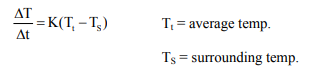# A body takes 4 min. to cool from 61° C to 59°C. If the temperature of the surroundings is 30°C,Question:

A body takes 4 min. to cool from 61° C to 59°C. If the temperature of the surroundings is 30°C, the time taken by the body to cool from 51°C to 49° C is :

1. 4 min.

2. 3 min.

3. 8 min.

4. 6 min.

Correct Option: , 4

Solution:$\frac{61-59}{4}=\mathrm{K}\left(\frac{61+59}{2}-30\right)$     .......(1)

$\frac{51-49}{t}=K\left(\frac{51+49}{2}-30\right)$          ......(2)

Divide (1) & (2)

$\frac{t}{4}=\frac{60-30}{50-30}=\frac{30}{20}$

so, $\mathrm{t}=6$ minutes